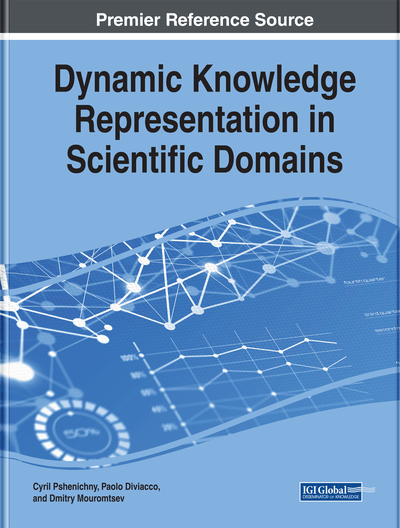# Qualitative and Quantitative Formalisms for Knowledge Representation in the Theory of Multitudes

Cyril Pshenichny (ITMO University, Russia)
DOI: 10.4018/978-1-5225-5261-1.ch002

## Abstract

The theory of multitudes pretends to be an alternative to virtually all existing versions of the set theory and claims to better handle the knowledge about changing and evolving world. Then, by analogy, one may expect an original logical system based on the theory of multitudes, and within this logic, an authentic calculus. This chapter presents such calculus. Moreover, a new mathematical methodology can be developed on top of it, which together with the underlying logic, should clearly separate qualitative and quantitative, static and dynamic concerns and offer a formal method to proceed from representation of expert knowledge to modeling the world this knowledge is about.
Chapter Preview
Top

## Introduction

Knowledge engineering implies not only extraction of knowledge from experts and putting into a computer-tractable form, but also making it complete and self-consistent. The latter task necessarily requires inference, which seems to be the only tool to fulfill it. For the traditional (static) knowledge engineering, this tool has been logical calculus, either in its strong (i.e., sensu Gentzen, 1934) or weak (simple modus ponens or modus tollens) form. However, the classical logic is indivisible from the classical set theory, which, as was shown above (Pshenichny, 2017, see Chapter 1 of this book), is crucially different from the theory of multitudes. Therefore, another formalism is needed to provide an inference based on the theory of multitudes. The purpose of the present work is to suggest such a formalism.

To achieve this purpose, we will:

• Consider an opportunity of creation of a calculus within the theory of multitudes;

• If a calculus can be created, find out whether it may be efficient at least for some cases;

• Discuss what should be done to extend these cases to known and possible methods of dynamic knowledge engineering.

Top

## The Novorossian Logic

Let us denote the Novorossian logic as a formal system, which includes

• 1.

The language that has:

• a.

Meanings: S, P and E and relations: “~”, ““, “” and “=”.

• b.

Technical signs: left bracket “(“, right bracket “)”, comma “,” order subscript indices, 1, 2, …, i, n, and dots, “”;

• c.

Metasymbols: possible negation, “(~)”, lists of primetas (Greek capitals Α, Β, Γ, Δ, etc.), universal meaning of thing, V, and exclusion sign EXCL;

• d.

The truth values: truth (T) and its opposite false (F);

• e.

The turnstile: or horizontal line;

• f.

Indicators of disclosure, *, of reference, , of being universum, u;

• 2.

The formulae: complete and incomplete;

• 3.

The calculus of meanings and

• 4.

Nothing else.

Meanings and relations have been defined by Pshenichny (2017; see previous chapter). Technical signs are not defined.

Metasymbols include possible negation (“with or without negation”), “(~)”, lists of primetas (Greek capitals Α, Β, Γ, Δ, etc.), universal meaning of thing, V, and an exclusion sign EXCL. Possible negation and exclusion sign have been introduced in the quoted chapter. The list of primetas Δi can be inductively defined asΔ1=(~)P1 if i=1;Δ2=(~)P1, (~)P2 if i=2;Δi=(~)P1, (~)P2, …, (~)Pi if i>2.List of universa is:Γ1= Pu1 if i=1;Γ2= Pu1, Pu2 if i=2;Γi= Pu1, Pu2, …, Pui if i>2.Universal meaning of thing, V (standing for “vesch”, the Russian for “thing”) is:

## Complete Chapter List

Search this Book:
Reset